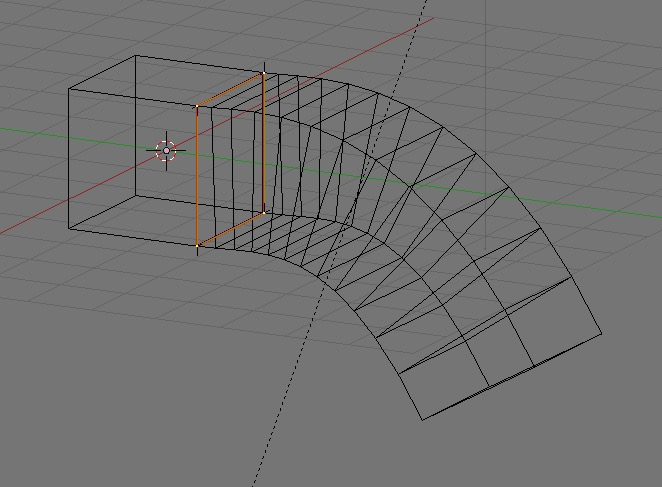# Extrude along a curve not working

The author uses a cylinder and a bezier curve but when I use a cube it doesn’t work, I get a flat object. I tried applying rotation/scale/location and changing the origin but nothing works.The author does the following:

• ``````Create a cylinder (no caps)
``````
• ``````Rotate it 90 degrees about the X.
``````
• ``````Scale it up a bit in the Y.
``````
• ``````On the positive Y end, select an edge loop.
``````
• ``````Shift + S: -&gt; cursor to selected
``````
• ``````Go to top view.
``````
• ``````Create a Bezier curve.
``````
• ``````Rotate the Bezier curve 90 degrees.
``````
• ``````Scale curve in the X to make it completely straight. (I just moved the control point handle instead)
``````
• ``````Select last control point on curve in negative Y direction. (Not handle)
``````
• ``````Shift + S: -&gt; Selection to Cursor
``````
• ``````In the positive Y direction, select some control points on the curve, move/rotate them in the -Z    direction to create a 90 degree arc.
``````
• ``````Go to the positive Y end of the cylinder and select an edge loop.
``````
• ``````Duplicate it.
``````
• ``````Convert it to a curve.
``````
• ``````Go to the Geometry tab of the Bezier curve and select the duplicated edeg loop.
``````
• ``````In the "Bevel Object" drop down select the edge loop you converted to a curve.
``````
• ``The duplicated edge loop will be extruded along the curve.``

Did you rotate your cube by 90 degrees in object mode as shown in the video with the cylinder and not in edit mode.

In this case the object needs to have the rotation so the face created is in the correct orientationWhen you add a curve circle for use as a bevel object it gets added pointing in the global Z axis, this is the correct alignment that you need to match, hence the need for the rotation